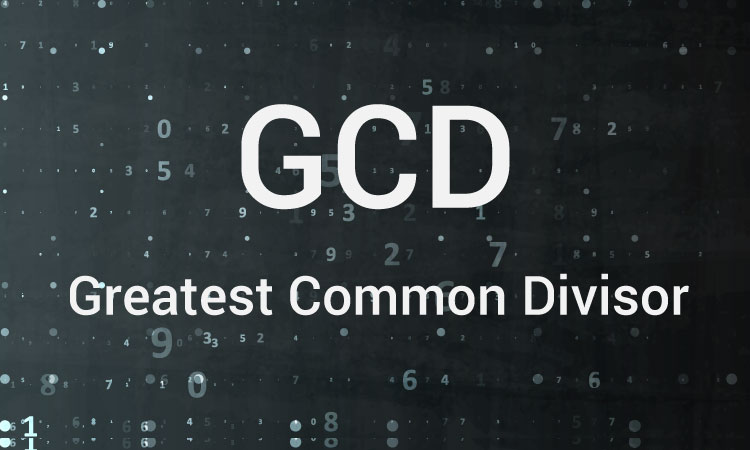# C Program to Find GCD of two Numbers

Examples on different ways to calculate GCD of two integers (for both positive and negative integers) using loops and decision making statements.To understand this example, you should have the knowledge of the following C programming topics:

The HCF or GCD of two integers is the largest integer that can exactly divide both numbers (without a remainder).

There are many ways to find the greatest common divisor in C programming.

## Example #1: GCD Using for loop and if Statement

``````#include <stdio.h>
int main()
{
int n1, n2, i, gcd;

printf("Enter two integers: ");
scanf("%d %d", &n1, &n2);

for(i=1; i <= n1 && i <= n2; ++i)
{
// Checks if i is factor of both integers
if(n1%i==0 && n2%i==0)
gcd = i;
}

printf("G.C.D of %d and %d is %d", n1, n2, gcd);

return 0;
}```
```

In this program, two integers entered by the user are stored in variable n1 and n2.Then, `for` loop is iterated until i is less than n1 and n2.

In each iteration, if both n1 and n2 are exactly divisible by i, the value of i is assigned to gcd.

When the `for` loop is completed, the greatest common divisor of two numbers is stored in variable gcd.

## Example #2: GCD Using while loop and if...else Statement

``````#include <stdio.h>
int main()
{
int n1, n2;

printf("Enter two positive integers: ");
scanf("%d %d",&n1,&n2);

while(n1!=n2)
{
if(n1 > n2)
n1 -= n2;
else
n2 -= n1;
}
printf("GCD = %d",n1);

return 0;
}``````

Output

```Enter two positive integers: 81
153
GCD = 9```

This is a better way to find the GCD. In this method, smaller integer is subtracted from the larger integer, and the result is assigned to the variable holding larger integer. This process is continued until n1 and n2 are equal.

The above two programs works as intended only if the user enters positive integers. Here's a little modification of the second example to find the GCD for both positive and negative integers.

## Example #3: GCD for both positive and negative numbers

``````#include <stdio.h>
int main()
{
int n1, n2;

printf("Enter two integers: ");
scanf("%d %d",&n1,&n2);

// if user enters negative number, sign of the number is changed to positive
n1 = ( n1 > 0) ? n1 : -n1;
n2 = ( n2 > 0) ? n2 : -n2;

while(n1!=n2)
{
if(n1 > n2)
n1 -= n2;
else
n2 -= n1;
}
printf("GCD = %d",n1);

return 0;
}``````

Output

```Enter two integers: 81
-153
GCD = 9```

You can also use recursion to find the GCD.